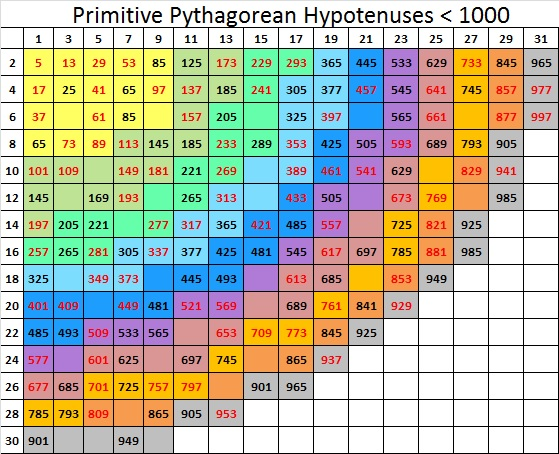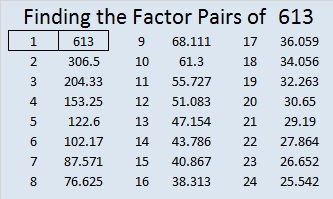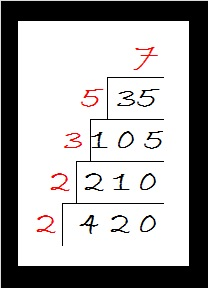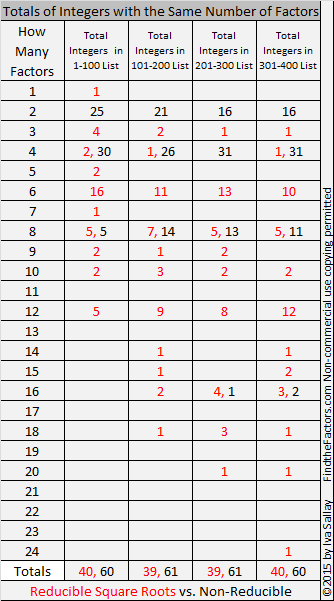# 1627 Color-Coded Prime Numbers

### Today’s Puzzle:

Study this color-coded chart of prime numbers. 1627 is the smallest prime number that begins something special. Can you figure out what that is?Also, why do you think I’ve underlined some of the other prime numbers on the list?

Memorizing which numbers are prime can be a big time-saver in mathematics. How many in a row can you recite without looking?

### Factors of 1627:

• 1627 is a prime number.
• Prime factorization: 1627 is prime.
• 1627 has no exponents greater than 1 in its prime factorization, so √1627 cannot be simplified.
• The exponent in the prime factorization is 1. Adding one to that exponent we get (1 + 1) = 2. Therefore 1627 has exactly 2 factors.
• The factors of 1627 are outlined with their factor pair partners in the graphic below.How do we know that 1627 is a prime number? If 1627 were not a prime number, then it would be divisible by at least one prime number less than or equal to √1627. Since 1627 cannot be divided evenly by 2, 3, 5, 7, 11, 13, 17, 19, 23, 29, 31, or 37, we know that 1627 is a prime number.

### More about the Number 1627:

1627 is the sum of two consecutive numbers:
813 + 814 = 1627.

1627 is also the difference of two consecutive squares:
814² – 813² = 1627.

What do you think of that?

# I can tell that 613 is a prime number just by looking at it!

613 is the 18th centered square number because 17 and 18 are consecutive numbers and 18² + 17² = 613.613 is the hypotenuse of the primitive Pythagorean triple 35-612-613, which was calculated using (18^2) – (17^2), 2 x 17 x 18, and (18^2) + (17^2).

Knowing that (18^2) + (17^2) = 613, I can tell that 613 is a prime number just by looking at it! Here’s what I see:

• 613 obviously is not divisible by 5.
• It is almost as obvious that 613 is not divisible by 13.
• 18^2 is not divisible by 17 so (18^2) + (17^2) is not divisible by 17. Thus 613 is not divisible by 17.
• Since 613 is not divisible by 5, 13, or 17, it is a prime number.

“What?” you say. What about all the other possible prime factors less than 24.8, the approximate value of √613? Don’t we need to TRY to divide 613 by 2, 3, 7, 11, 19, and 23?

No, we don’t. Those numbers don’t matter at all. Since 17^2 + 18^2 = 613, and because 17 and 18 have NO common prime factors and only one of them is odd, I already know that none of those numbers will divide into 613. Let me show you what I mean.

Look at this chart of ALL the primitive Pythagorean triple hypotenuses less than 1000:The numbers in red are all prime numbers and appear on the chart only one time. Every prime number less than 1000 that can be written in the form 4N + 1 appears somewhere on the chart. 613 can be found at the intersection of the column under 17 and the row labeled 18.

The black numbers on the colorful squares are composite numbers and many of them appear on the chart above more than one time. There is something very interesting about the prime factors of these composite numbers. Every single one of those factors is also the hypotenuse of a primitive Pythagorean triple! Also notice that at least one of those factors is less than or equal to the square root of the composite number. Here is a chart of those composite numbers, how many times they appear on the chart above, and their prime factorizations:Also notice that if you divide any of the above composite numbers OR their factors by 4, the remainder is always 1.

I have demonstrated that the following is true for any integer less than 1000. I wasn’t sure if it had been proven for integers in general so I asked Gordan Savin, a professor at the University of Utah, who teaches number theory. He informed me that it has been proven and that it follows from the uniqueness of factorization of Gaussian integers.

In conclusion, if an integer can be written as the sum of two squares, one odd and one even, and the numbers being squared have no common prime factors, then ALL the factors of that odd integer will be hypotenuses of primitive Pythagorean triples. If the integer is a composite number, at least one of those primitive hypotenuses will be less than or equal to the square root of that integer.

Here’s the same thing in more standard mathematical language:

If a natural number of the form 4N + 1 can be written as the sum of two squares (a^2)+ (b^2) and if a and b have no common prime factors, then ALL the factors of 4N + 1 will be of the form 4n + 1. If 4N + 1 is a composite number, there will exist at least one prime factor, 4n + 1 ≤  √(4N + 1). If no such factor exists, then 4N + 1 is a prime number.

• 613 is a prime number.
• Prime factorization: 613 is prime.
• The exponent of prime number 613 is 1. Adding 1 to that exponent we get (1 + 1) = 2. Therefore 613 has exactly 2 factors.
• Factors of 613: 1, 613
• Factor pairs: 613 = 1 x 613
• 613 has no square factors that allow its square root to be simplified. √613 ≈ 24.7588How do we know that 613 is a prime number? If 613 were not a prime number, then it would be divisible by at least one prime number less than or equal to √613 ≈ 24.8. Since 613 cannot be divided evenly by 2, 3, 5, 7, 11, 13, 17, 19, or 23, we know that 613 is a prime number. But as I explained above since (18^2) + (17^2) = 613, and consecutive integers 18 and 17 have no common prime factors, it is only necessary to check to see if 613 is divisible by 5, 13, or 17. Since it isn’t divisible by any of those three numbers, 613 is a prime number.

# 546 and Level 3

### A Math Vocabulary Puzzle:

Puzzles can be a great resource in teaching mathematics. I’ve made crossword puzzles to review vocabulary words before, but none of them looked as inviting to complete as the one Resourceaholic recommended in her 7 July 2015 post on end of term resources. What a difference a little clip art makes to a well-constructed crossword puzzle! The post also includes links to several other amazing mathematics-related puzzles including a polygon word search and a number sequence number search puzzle. Check it out!

### Today’s Puzzle:Print the puzzles or type the solution on this excel file: 10 Factors 2015-07-06

A Logical Approach to solve a FIND THE FACTORS puzzle: Find the column or row with two clues and find their common factor. Write the corresponding factors in the factor column (1st column) and factor row (top row).  Because this is a level three puzzle, you have now written a factor at the top of the factor column. Continue to work from the top of the factor column to the bottom, finding factors and filling in the factor column and the factor row one cell at a time as you go.### Factors of 546:

• 546 is a composite number.
• Prime factorization: 546 = 2 x 3 x 7 x 13
• The exponents in the prime factorization are 1, 1, 1, and 1. Adding one to each and multiplying we get (1 + 1)(1 + 1)(1 + 1)(1 + 1) = 2 x 2 x 2 x 2 = 16. Therefore 546 has exactly 16 factors.
• Factors of 546: 1, 2, 3, 6, 7, 13, 14, 21, 26, 39, 42, 78, 91, 182, 273, 546
• Factor pairs: 546 = 1 x 546, 2 x 273, 3 x 182, 6 x 91, 7 x 78, 13 x 42, 14 x 39, or 21 x 26
• 546 has no square factors that allow its square root to be simplified. √546 ≈ 23.36664### Sum-Difference Puzzle:

546 has eight factor pairs. One of the factor pairs adds up to 85, and a different one subtracts to 85. If you can identify those factor pairs, then you can solve this puzzle!### More about the Number 546:

546 is made from 3 consecutive numbers so it is divisible by 3.

The sum of all the prime numbers from 53 to 83 is 546. Can you list all those prime numbers?

546 is the hypotenuse of the Pythagorean triple 210-504-546. Those three numbers have eight common factors, but what is the greatest common factor?

# 420 Factor Trees

Did you know that the sum of all the prime numbers between 100 and 110 equals 420? Yes, 101 + 103 + 107 + 109 = 420.

Since 20 × 21 = 420, we know that 420 is the sum of the first 20 EVEN numbers. Thus,

• 2 + 4 + 6 + 8 + 10 + 12 + 14 + 16 + 18 + 20 + 22 + 24 + 26 + 28 + 30 + 32 + 34 + 36 + 38 + 40 = 420.

420 is the smallest number that can be divided evenly by all the natural numbers from 1 to 7.

420 has a LOT of factors, more than most people would think it does.  In fact, of all of the numbers from 1 to 420, there is only one number, 360, that has as many factors as 420 has.

There are 4 different prime numbers that can divide evenly into 420. Here are those factor trees:Many, but not all, of the factors of 420 are listed somewhere on those four trees.

• 420 is a composite number.
• Prime factorization: 420 = 2 x 2 x 3 x 5 x 7, which can be written 420 = (2^2) x 3 x 5 x 7
• The exponents in the prime factorization are 2, 1, 1, and 1. Adding one to each and multiplying we get (2 + 1)(1 + 1)(1 + 1)(1 + 1) = 3 x 2 x 2 x 2 = 24. Therefore 420 has exactly 24 factors.
• Factors of 420: 1, 2, 3, 4, 5, 6, 7, 10, 12, 14, 15, 20, 21, 28, 30, 35, 42, 60, 70, 84, 105, 140, 210, 420
• Factor pairs: 420 = 1 x 420, 2 x 210, 3 x 140, 4 x 105, 5 x 84, 6 x 70, 7 x 60, 10 x 42, 12 x 35, 14 x 30, 15 x 28, or 20 x 21
• Taking the factor pair with the largest square number factor, we get √420 = (√4)(√105) = 2√105 ≈ 20.4939Each factor pair, except 1 x 420, can make its own factor tree. Here are some factor trees featuring the other seven factor pairs:If I hadn’t made all the prime numbers in red, it’s possible that one or more of the prime numbers might get forgotten. That is why I prefer the cake method for finding the prime factorization of a number. All of the prime numbers are listed in numerical order on the outside of the cake.# 40% of Numbers Up To 400 Have Square Roots That Can Be Simplified

• 400 is a composite number.
• Prime factorization: 400 = 2 x 2 x 2 x 2 x 5 x 5, which can be written 400 = (2^4) x (5^2)
• The exponents in the prime factorization are 4 and 2. Adding one to each and multiplying we get (4 + 1)(2 + 1) = 5 x 3 = 15. Therefore 400 has exactly 15 factors.
• Factors of 400: 1, 2, 4, 5, 8, 10, 16, 20, 25, 40, 50, 80, 100, 200, 400
• Factor pairs: 400 = 1 x 400, 2 x 200, 4 x 100, 5 x 80, 8 x 50, 10 x 40, 16 x 25, or 20 x 20
• 400 is a perfect square. √400 = 20A few months ago I made a chart showing the number of factors for the first 300 counting numbers. Since this is my 400th post, I’d like to include a chart showing the number of factors for all the numbers from 301 to 400. I’m also interested in consecutive numbers with the same number of factors and whether or not the square root of a number can be reduced. The red numbers have square roots that can be reduced.The longest streak of consecutive numbers with the same number of factors is only three. There are three sets of three consecutive numbers on this chart. (Between 200 and 300 there was a streak of four consecutive numbers with six factors each.)

How do the number of factors of these 100 numbers stack up against the previous 300? The following chart shows the number of integers with a specific number of factors and how many of those integers have reducible square roots:• 39.5% or slightly less than 40% of the numbers up to 400 have reducible square roots.
• Most of these numbers have 2, 4, or 8 factors. Numbers with two factors are prime numbers. Almost all numbers with four factors are the product of two different prime numbers, and nearly two-thirds of the numbers with eight factors are the product of three different prime numbers.
• There isn’t much change between the percentages of reducible square roots from one list to the next.